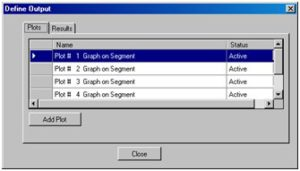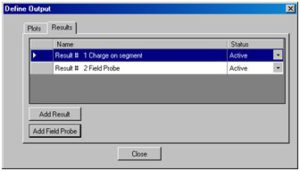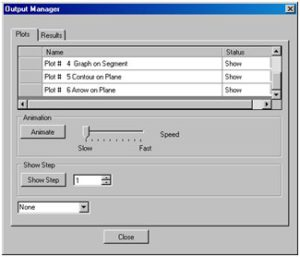# Managing Multiple Results with the New Output Manager

INTEGRATED’s applications are moving more toward providing analysis from multiple solutions, such as transient analysis and time domain analysis. For these types of applications, users will feel the need to define the kinds of calculation and output they want to see at each step before generating the solutions. This will make generating useable results work more smoothly and quickly once the analysis is finished. INTEGRATED is, therefore, introducing a new interface for pre-defining plots and calculations to be performed after each solution step and an interface to manage and plot these outputs using animations, step-through plots and graphs of calculated values.

The process of working with multiple step plots and calculations involves two steps.

The pre-solving step is the Define Output process, where plots and results are defined. This will instruct the application which calculations will be performed after each solution step. Users will pre-define the output through the Define Output interface:The Plots tab on this dialog is for defining the plots (contours, arrows, etc.) to be generated at each solution step. Selecting “Add Plot” allows you to define a new plot. Plot Properties are set in a tabbed dialog, designed to let you find the kind of plot you want more easily and intuitively.

The Results tab allows you to define single value calculations such as forces on parts, power loss on structures, or the field values at some point in space.Many different plots and results can be defined and then activated or deactivated to save time in the overall analysis. Each plot and result is named for easy reference.After solving, all of the data for the requested plots and results are accumulated. You can then use the Output Manager to generate animations of multiple plots or step through the plots over the range of the transient analysis.

You can also use the Output Manager to generate graphs of single value calculations against the transient time step or display a table of the results for the analysis.